# sklearn.datasets.make_blobs¶

sklearn.datasets.make_blobs(n_samples=100, n_features=2, centers=None, cluster_std=1.0, center_box=(-10.0, 10.0), shuffle=True, random_state=None)[source]

Generate isotropic Gaussian blobs for clustering.

Read more in the User Guide.

Parameters: n_samples : int or array-like, optional (default=100) If int, it is the total number of points equally divided among clusters. If array-like, each element of the sequence indicates the number of samples per cluster. n_features : int, optional (default=2) The number of features for each sample. centers : int or array of shape [n_centers, n_features], optional (default=None) The number of centers to generate, or the fixed center locations. If n_samples is an int and centers is None, 3 centers are generated. If n_samples is array-like, centers must be either None or an array of length equal to the length of n_samples. cluster_std : float or sequence of floats, optional (default=1.0) The standard deviation of the clusters. center_box : pair of floats (min, max), optional (default=(-10.0, 10.0)) The bounding box for each cluster center when centers are generated at random. shuffle : boolean, optional (default=True) Shuffle the samples. random_state : int, RandomState instance or None (default) Determines random number generation for dataset creation. Pass an int for reproducible output across multiple function calls. See Glossary. X : array of shape [n_samples, n_features] The generated samples. y : array of shape [n_samples] The integer labels for cluster membership of each sample.

make_classification
a more intricate variant

Examples

>>> from sklearn.datasets.samples_generator import make_blobs
>>> X, y = make_blobs(n_samples=10, centers=3, n_features=2,
...                   random_state=0)
>>> print(X.shape)
(10, 2)
>>> y
array([0, 0, 1, 0, 2, 2, 2, 1, 1, 0])
>>> X, y = make_blobs(n_samples=[3, 3, 4], centers=None, n_features=2,
...                   random_state=0)
>>> print(X.shape)
(10, 2)
>>> y
array([0, 1, 2, 0, 2, 2, 2, 1, 1, 0])


## Examples using sklearn.datasets.make_blobs¶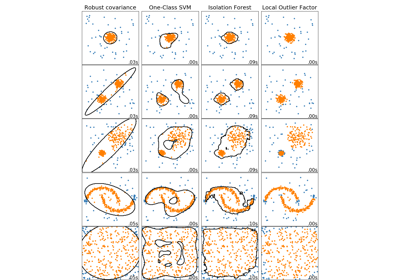Comparing anomaly detection algorithms for outlier detection on toy datasets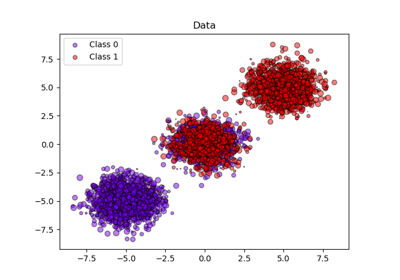Probability calibration of classifiers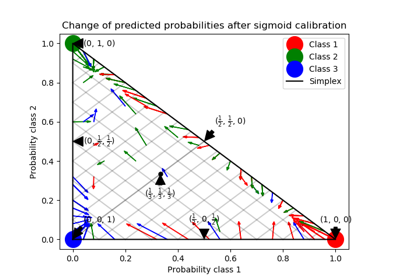Probability Calibration for 3-class classification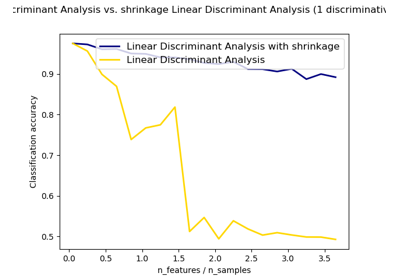Normal and Shrinkage Linear Discriminant Analysis for classification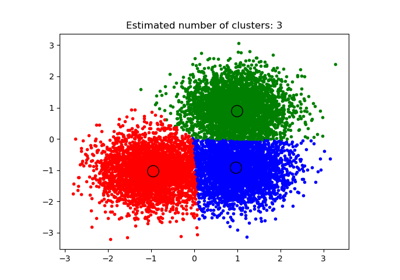A demo of the mean-shift clustering algorithm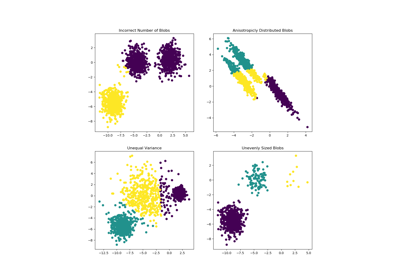Demonstration of k-means assumptions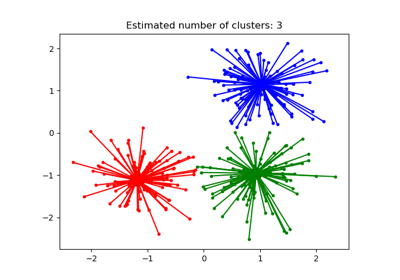Demo of affinity propagation clustering algorithm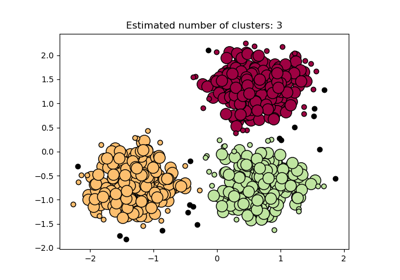Demo of DBSCAN clustering algorithm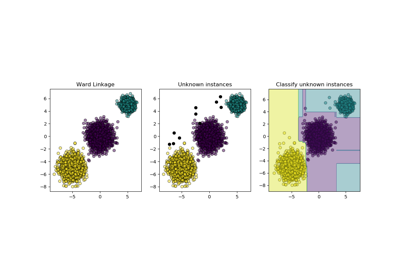Inductive Clustering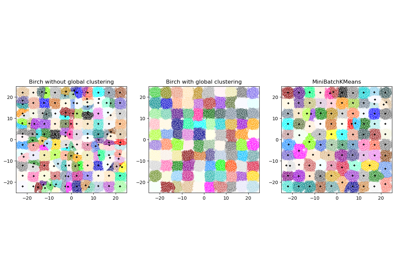Compare BIRCH and MiniBatchKMeans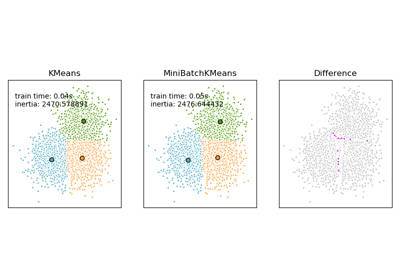Comparison of the K-Means and MiniBatchKMeans clustering algorithms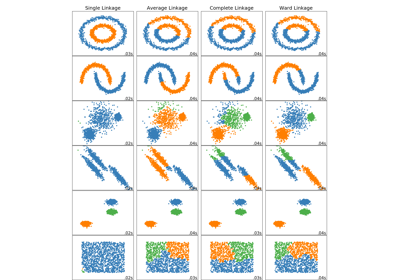Comparing different hierarchical linkage methods on toy datasets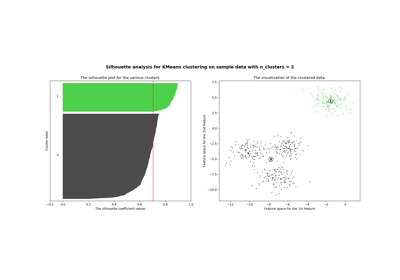Selecting the number of clusters with silhouette analysis on KMeans clustering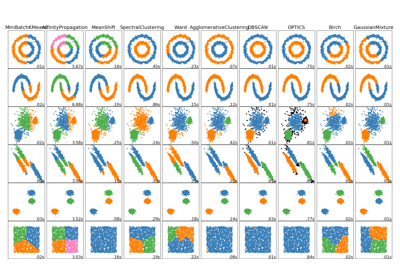Comparing different clustering algorithms on toy datasets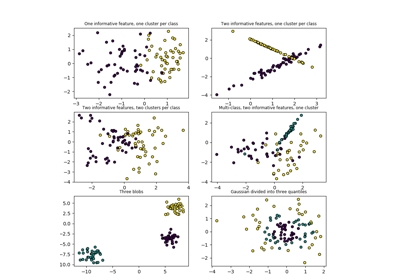Plot randomly generated classification dataset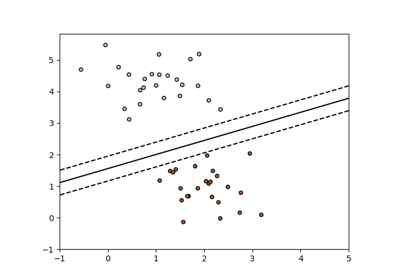SGD: Maximum margin separating hyperplane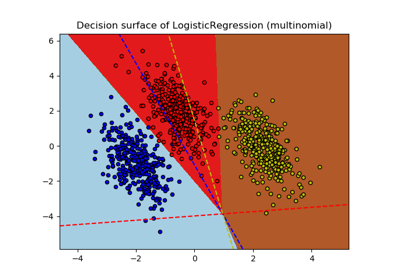Plot multinomial and One-vs-Rest Logistic Regression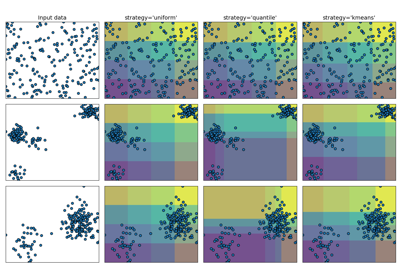Demonstrating the different strategies of KBinsDiscretizer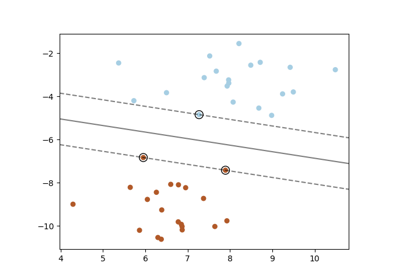SVM: Maximum margin separating hyperplane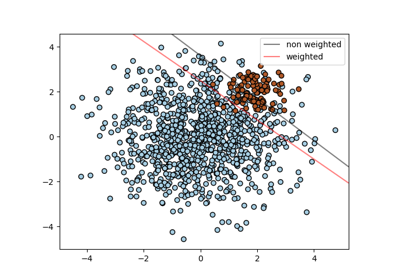SVM: Separating hyperplane for unbalanced classes